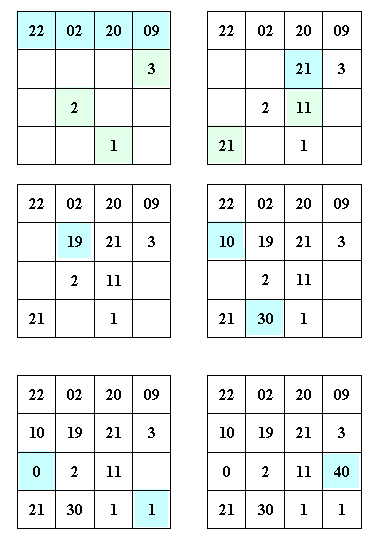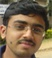# Sunday Pastime #29: Birthday Magic Square/ Date Magic Square

#### Written by Pavan Kumar on February 22, 2009

It has been long, really long, I made you wait for 2 months after I promised you about date magic square. Here is the step by step procedure for that. The steps are very easy to follow and you can create on birthday magic square or date magic square in 1 minute, you don’t need to be a genius in maths to do this.1. On the first row, write down the date of interest. I have considered that of today 22-02-2009. You can consider in any format, but split the year into two. Write any three consecutive numbers the way I have written.
2. Calculate the magic constant (sum of elements of each row, column, diagonal) by summing up the elements of first row. In this case, it is S=22+2+20+9 = 53. Now, calculate the element in row2, col3 by subtracting the other 3 elements of that sub-square by magic constant. 53 – (20+9+3) = 21. Now, you see, column 3 has only one blank space which can be easily filled by subtracting other elements from magic constant. 53-(20+21+1) = 11. Also, the left bottom corner can be filled by subtracting other elements of that diagonal from magic constant. 53-(9+21+2)=21.
3. Follow the same way to complete the row 2, column 2 element from central sub-square. 53-(21+11+2)=19.
4. By this time, you know, how to complete 2nd row and 2nd column completely.
5. Next, fill 1st column and 4th row.
6. Finally left is only one blank space, complete it the same way.

This is one of the very rare things one expects on a special occasions, you can prepare one by yourself and present the same to your beloved during any special occasions in different ways like getting it laminated, making its animation, printing it on cups, t-shirts and many more.

People who liked this also read:

Category: entertainment